Legacy application – Refactoring or reengineering? (VI)In the two previous posts, we presented the definition of characterization tests as proposed by Michael Feathers in his book “Working Effectively with Legacy Code”.

We showed briefly how we can use such tests in order to acquire the knowledge of the application’s behavior. I say briefly because, ideally, we should have developped and presented some tests as examples, but that would require several posts, and this series is already very long. Just have a look to Michael Feathers book if you want to go more in depth into this.

We just have to remember that these tests will facilitate the transfer of knowledge from our Legacy application (Microsoft Word 1.1a), and any subsequent refactoring or reengineering operation will be faster and safer.

Test coverage

What should be the scope of this operation of ‘characterization’? When can we consider that our test coverage is sufficient, and start making changes in the code? Is it possible to quantify the effort it represents?

Michael Feathers recommends writing as many tests as we consider necessary for each block of code that we will have to change in the future. However, what if no change is planned in the future?

This happen a lot of times: when a company buys a software publisher, it may not wish to change its product, but simply provide support until the software dies a natural death, when no more customer pays for the maintenance.
Another case I know: IT departments that have almost completely lost the knowledge of entire groups of Cobol applications, PL1, Natural, Oracle Forms … These applications are:

• Often critical because in the historical heart of the information system.
• Fully tested, so don’t have much defects and corrective maintenance.
• Evolve very little, and rather at their interfaces, in order to allow new applications to connect with them.

One possible strategy for these IT departments is to outsource the code, but paying attention to the transfer of knowledge, in order to avoid to break an application that works.

The mission assigned to us is to calculate the cost of knowledge tranfer to another team. How can we estimate the effort to discover the code through these characterization tests? Is there a formula that could help us to assess this effort and plan for resources and a calendar?

I always consider in the various audits that I do, that Cyclomatic Complexity is representative of the effort of tests.

A small, recent application, not critical, used within the company and without external users (intranet for instance), with about 6,000 points of CC, can be validated with unit and integration testing done by the project team, without a formalized QA phase.

An old application, open to the public – for example, a ‘Customers’ front-end to various other applications and business solutions for Orders, Invoicing, Inventory, etc. – thus critical for the company, and with more than 60,000 points of CC: QA phase required by a team of specialized testers with formalized test cases, and if possible automated.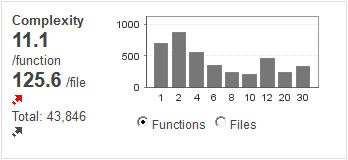We have seen that our application has 43 846 CC points, distributed in 3 936 functions and 349 files.

The distribution of the Cyclomatic Complexity among these functions  is as following:

Table 1 – Cyclomatic Complexity of the functions (Word 1.1a)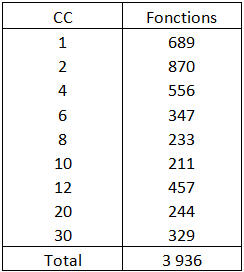I will not have an objective of a test coverage of 100% of the Cyclomatic Complexity, because as you probably know, beyond a certain limit, the time to write new tests is becoming longer. The test effort obeys (approximately) to some kind of a Pareto distribution, so that it would be possible to write 80% of the tests in 20% of the time.

In fact, I think 80/20 is a bit optimistic and I’m considering that 60% of the tests can be performed in 50% of the time, and an additional 40% will require another 50% of the total effort. Our main goal is to first perform a knowledge transfer to a new team, not to achieve a test coverage of 100%.

However, the most complex functions require an increased attention because they have a higher risk of introducing a default during a change. These functions are also strong candidates for a refactoring, so to a better ‘characterization’, especially if they are difficult to read, with a high number of lines of code or defects affecting their maintainability. We will therefore increase our requirements for the tests of these functions.

Therefore, I will have the following assumptions:

• Functions with a CC lower or equal to 20 points will have a test coverage of 60% of the Cyclomatic Complexity.
• For functions with more than 20 points of CC, we want a 100% coverage of the Cyclomatic Complexity.

SonarQube has a rule ‘Avoid too complex function’ which lists the functions beyond 20 points of CC, with their exact number. This allowed us to calculate the following distribution:

Table 2 – Distribution of the most complex functions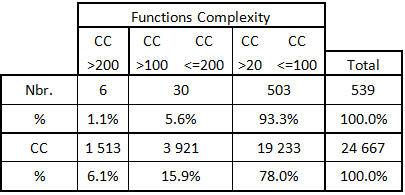Another rule ‘Function/method should not have too many lines’ lists also the functions with more than 100 lines of code, and their exact number.
So I can cross these two lists to identify functions with over 20 points of CC and more than 100 lines of code. I get the following distribution:

Table 3 – Distribution of the most complex functions by size (LOC)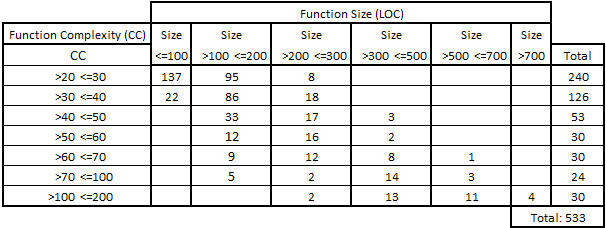I dit not include in the table above the 6 most complex functions beyond 200 CC points, which are also found in complex programs or with a large number of lines:

Table 4 – 6 programs with the most complex functionsEstimation of the test effort

So we get a classification of the different components into three categories, from the simplest functions with few lines to complex functions of large to very large size, and also an high number of violations to good programming practices that can be harmful when trying to understand the code.

To calculate a measure of the test effort, I will use the following formula:

Test Effort = Code Reading Time + Characterization Test

and: Characterization Test = CC x N (mn)

I use three variables in this formula:

• Code Reading (CR) time is the time required to ‘read’ a function and define the corresponding characterization tests.
• Characterization Test (CT) is the time for writing and executing the tests, with N a number of minutes depending on the Cyclomatic Complexity, that I will adapt depending on the type of component.

Let’s remember that a characterization test is used to describe the behavior of a block of code and therefore, unlike a unit test or a regression test, does not try to verify that the code behaves correctly. A completely full understanding of the function and each of its variables, constants, parameters, and input/output values is not required. This is the reason why I say ‘read’ the function, that is to say, understand it not completely but enough to start writing characterization tests.

However, the more a function will be complex and difficult to read, with ‘goto’, ‘switch’, etc. the least it will be easy to understand. So I will use a factor of readability – Code Reading (CR) – to modulate the time needed to decipher the function.

I will also adjust the time of realization of the characterization tests, because it will be different depending on the number of points of CC. We saw in the last post that the most complex function of our application included ‘switch’ with conditions on several variables, rather easy to understand and test. In such a case, it will not take much more time to test a single ‘switch’ with 8 or 10 points of CC than a function with an ‘if … else’ representing 2 or 3 points of CC.

I will once again have the following assumptions:

• For functions with a CC equal or less than 20 points, the time of writing the tests will be of 4 minutes per point of CC.
• For functions with more than 20 points of CC, the time of writing tests will be equal to 2 minutes per point of CC.

With this formula, a function equal to a Cyclomatic Complexity of:

• 1, requires 30 seconds of reading time and 4 minutes of completion of the test(s), for a total of 4 minutes and 30 seconds.
• 2, requires 1 minute of reading time and 8 minutes of realizing tests for a total of 9 minutes.
• 8, requires 4 minutes of reading time and 32 minutes of performing tests for a total of 36 minutes.
• 12, requires 6 minutes of reading time and 48 minutes of writing tests for a total of 54 minutes.

In fact, I do not have the exact CC for functions with less than 20 points, so I’ll assume that the completion time of the tests will be 9 minutes for a function of 2-4 points of CC, 36 min for a function of 8-10 CC points, 54 min for a function between 12 and 20 points of CC, etc.

This implies a multiplier Readability Factor (RF%) equal to 1. I will modify the value of this factor when the functions will become more complex (beyond 20 points of CC) or less readable.

These figures seem realistic enough, or at least, do not seem understated. I can perfectly present these hypothesis to the project teams or the stakeholders: even if they understand that this is an approximation, this base seems acceptable to proceed with our estimation.

Let’s see what we get, first of all for the functions within 20 points of Cyclomatic Complexity:

Table 5 – Calculation of the test effort on the simplest functions (<20 CC)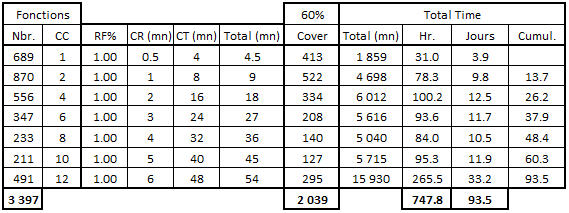We count 3 397 functions with less than 20 points of CC, for which we aim to cover 60% of the Cyclomatic Complexity, and therefore having tests equivalent to 2 039 functions. So:

• 413 of 689 functions with 1 point of CC, and a unit cost of test of 4.5 mn represent 31 hours of work or nearly 4 days (assuming 8 hours per day).
• 522 of 870 functions with 2-4 points of CC, and a unit cost of test of 9 minutes represent 78.3 hours or nearly 10 days, for a cumulative total (with the previous 4 days) of nearly 14 days.
• 295 of 491 functions with 12-20 points of CC, and a unit cost of test of 54 minutes represent approximately 33 working days, a third of the 93.5 days required in total for all these functions.

Let’s keep these numbers in mind for now and continue with more complex functions. We said that, for these functions:

• We want a test coverage equal to 100% of the Cyclomatic Complexity.
• We estimate the time of writing a characterization test equal to the number of CC points x 2 minutes.

• For less than 100 lines of code (LOC), RF% = 1
• For 100 to 200 LOC, RF% = 1.5
• For 200 to 300 LOC, RF% = 2
• For 300 to 500 LOC, RF% = 2.5
• For 500 to 700 LOC, RF% = 4
• Beyond 700 LOC (but less than 200 points of CC), RF% = 10. This concerns only four functions, not including the 6 most complex functions, which we will manage separately.

Here is the corresponding table:

Table 6 – Calculation of the test effort on very complex functions (20 <CC <200)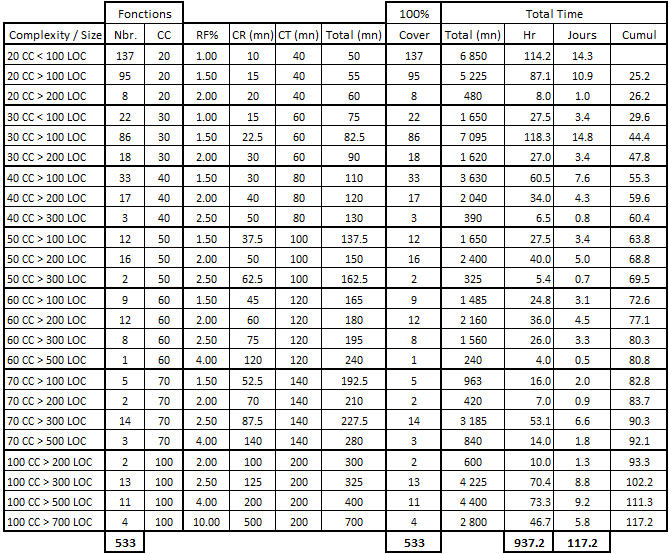Some explanations, in order to facilitate the understanding of this table:

• 137 functions with a CC between 20 and 30 points and less than 100 LOC, each representing a time of 40 minutes to write tests (RF% = 1) and a test coverage of 100% of the CC, require 14.3 days of work.
• 95 functions with a CC between 20 and 30 points and a size of 100 to 200 LOC, so with a Readibility Factor equal to 1.5 and a test effort of 55 minutes for each function, require 10.9 days of work. The cumulation with the previous job is equal to 14.3 + 10.9 = 25.2 days.
• 1 function with a CC between 60 and 70 points and a size between 500 and 700 LOC, thus with an RF% of 4, will need a Code Reading time of 120 minutes (60/2 x 4) and a Characterization Test time of 120 minutes also, for a total of 4 hours or a half day.
• 4 functions with a CC between 100 and 200 points and more than 700 LOC, with a RF% of 10, will have an estimated time of 700 minutes per function, for a total of nearly six days of work to ‘characterize’ these 4 functions.

I did a calculation specific to each of the 6 heaviest and most complex functions:

Table 7 – Estimation of the test effort on the 6 most complex functions (> 200 CC)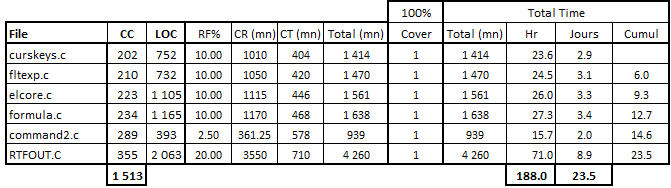Except the program ‘command2.c’ with a function of less than 400 lines, and therefore a RF% factor of 4, I assigned a RF% of 10 to other functions and an RF% of 20 for the most complex function in the program ‘RTFOUT.c’ (that we discussed in the previous post).

Synthesis

Based on the assumptions that we have chosen, we get a total of 234 days for the completion of characterization tests on our Legacy C application, with the goal of transferring the knowledge to another team, or during an outsourcing.

These 234 days, a little less than 12 man-months (based on 20 days per month) are composed of:

• 93.5 days for a test coverage of 60% of the total Cyclomatic Complexity for 3 397 functions with less than 20 points of CC.
• 117 days for a total coverage of the 533 functions between 20 and 200 points of CC.
• 23.5 days to characterize the 6 most complex functions.

What about these numbers? Are our assumptions correct or questionable? If we present these results to the project team or the management, what issues may arise and how to respond? What action plan to propose?